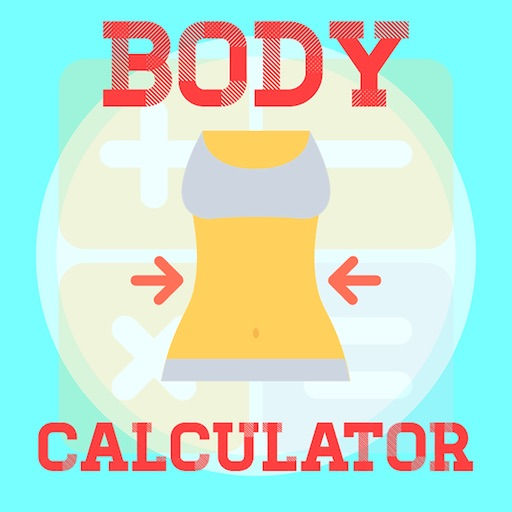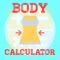## Why should you choose "Body Calculator"# Body Calculator Pro

by Putu Angga Risky RaharjaWhy should you choose "Body Calculator"?### App Details

Version
3.2
Rating
(2)
Size
36Mb
Genre
Health & Fitness Medical
Last updated
August 3, 2021
Release date
June 15, 2017

### App Screenshots### App Store Description

Why should you choose "Body Calculator"?
• Simple and very easy to use.
• Accurate and precise calculation.
• Both standard and metric measures can be used for calculation.
• Calculation and interpretation of body mass index (BMI).
• Calculation of body surface area (BSA) with 9 possible formulas.
• Calculation of ideal body weight (IBW) with 4 possible formulas.

"Body Calculator" is a medical calculator application to calculate body mass index (BMI), body surface area (BSA), and ideal body weight (IBW). Body mass index (BMI) is a simple index of weight-for-height. Body mass index (BMI) is commonly used to classify normal weight, underweight, overweight, and obesity in adults. Body mass index (BMI) is significantly correlated with body fat.

Body surface area (BSA) is the external surface area of the human body given in square meters. Body surface area (BSA) is a better indicator of metabolic mass than body weight because it is less affected by abnormal adipose mass. There are 9 formulas used to calculate body surface area (BSA) in this app, namely:
• Du Bois formula
• Fujimoto formula
• Gehan & George formula
• Haycock formula
• Mosteller formula
• Schlich formula, men
• Schlich formula, women
• Shuter & Aslani formula
• Takahira formula

"Body Calculator" app will also calculate your ideal body weight (IBW). The calculation of ideal body weight (IBW) depends on your height and gender. "Body Calculator" app has 5 most commonly used formulas to calculate ideal body weight (IBW), namely:
• Ideal body mass index (BMI)
• Robinson formula
• Miller formula
• Hamwi formula
• Devine formula